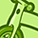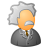cancel
Showing results for
Did you mean:Not applicable

## Incorporating if statements within variable expressions

Hello -

My data source has three columns:  Year, Country, Sales \$ (sales dollars by different countries from 2008-2012).  In QV I created two variables called "Start Year" and "End Year".  I then created an input box with with these two variables.  From an end-user perspective, the user enters a "start year" (2010 for example) and an "end year" (2012 for example).  The results are displayed in a straight table, where sales \$ are shown by country in "start year" and "end year" columns.  The expressions used to display are below:

Sum({\$<Year ={\$(vStartYear)}>}(Sales))

Sum({\$<Year ={\$(vEndYear)}>}(Sales))

I need to incorporate logic that says "if the year entered is less than 2012, divide sales by 1.3" (for either start or end year).  How do I do this?  I have other bar charts where I use the expression if (Year < 2012, (Sum (Sales)/1.3),(Sum (Sales)) successfully

(before anyone suggest that I just divide the sales totals by 1.3 in the source data, I'm simply using 1.3 as a proxy for this example.  In my actual QV solution, the "1.3" is a variable that can be changed by the user)

1 Solution

Accepted SolutionsMaster II

you can test the if condition using a text box first.

i text box

try: if (\$(vStartYear)< 2012,'YES','No')

if yes prints on text box that means the condition is working and then use the formula:

if (\$(vStartYear)< 2012, Sum({\$<Year ={\$(vStartYear)}>}Sales) / 1.3,

Sum({\$<Year ={\$(vStartYear)}>}Sales)

HTH

Sushil

3 RepliesCreator III

Hi,

Expr1:

if (\$(vStartYear)< 2012, Sum({\$<Year ={\$(vStartYear)}>}Sales) / \$(vFactor),

Sum({\$<Year ={\$(vStartYear)}>}Sales)

)

Expr2:

if (\$(vEndYear)< 2012, Sum({\$<Year ={\$(vEndYear)}>}Sales) / \$(vFactor),

Sum({\$<Year ={\$(vEndYear)}>}Sales)

)

vFactor = If(vStartYear < 2012 or vEndYear < 2012, 1.3, 1)

Regards,

somCreator II

Hi ,

You can evaluate the expression using date from which you have evaluate your year ,start year,end year...

if(\$(vEndYear)<=31-12-2012,sum({\$<date={">=\$(vStartYear)<=\$(vEndYear))"}>}Sales)/\$(vinput),sum({\$<date={">=\$(vStartYear)<=\$(vEndYear))"}>}Sales))

here vinput is the variable to divide total as per user input when year is less than 2012.

Regards,

UditMaster II

you can test the if condition using a text box first.

i text box

try: if (\$(vStartYear)< 2012,'YES','No')

if yes prints on text box that means the condition is working and then use the formula:

if (\$(vStartYear)< 2012, Sum({\$<Year ={\$(vStartYear)}>}Sales) / 1.3,

Sum({\$<Year ={\$(vStartYear)}>}Sales)

HTH

SushilCommunity Browser